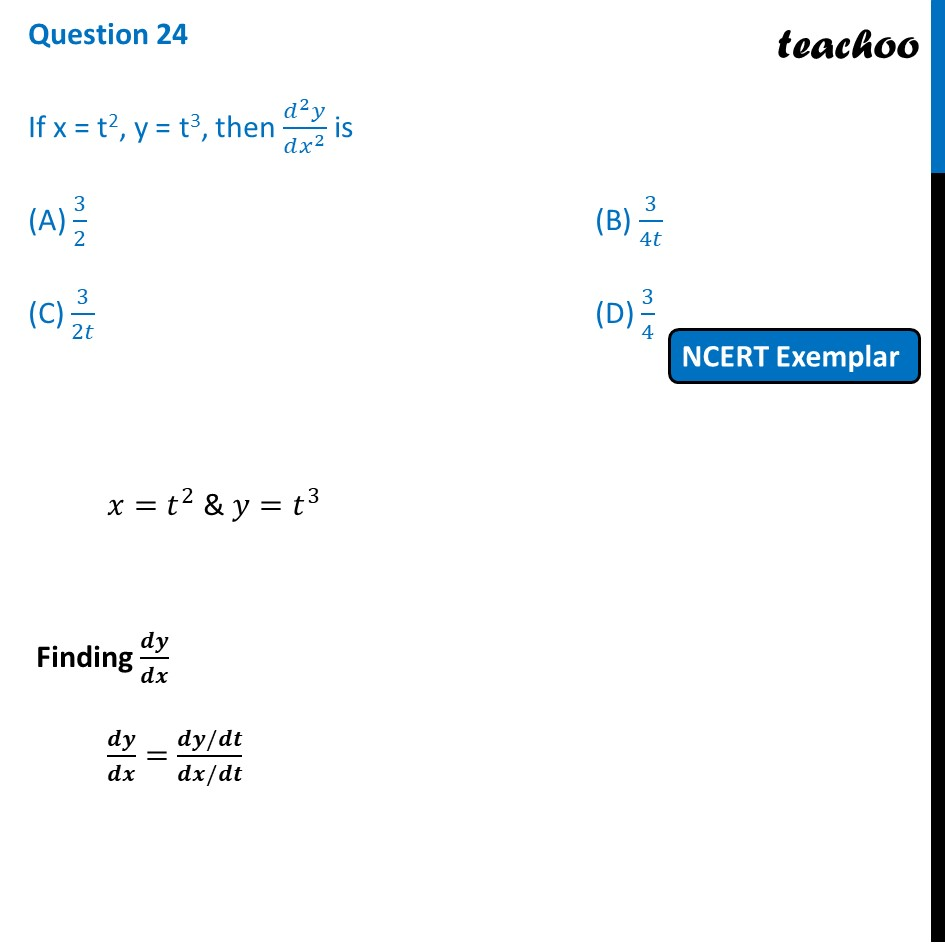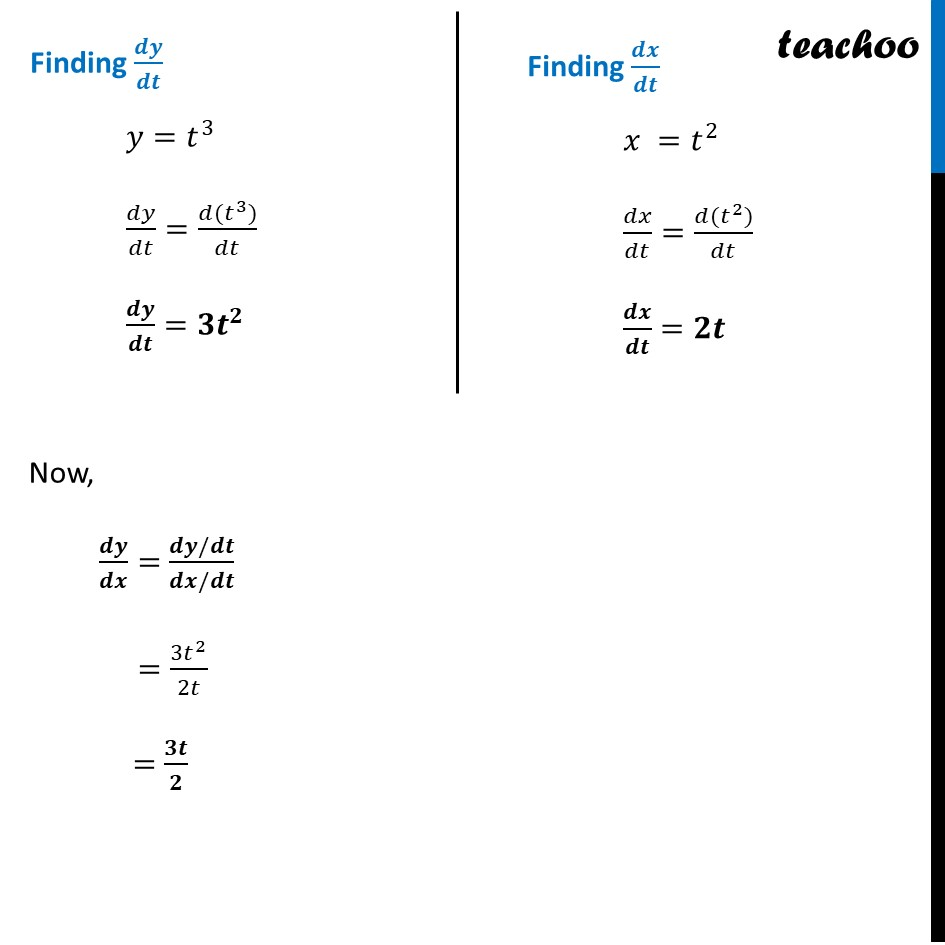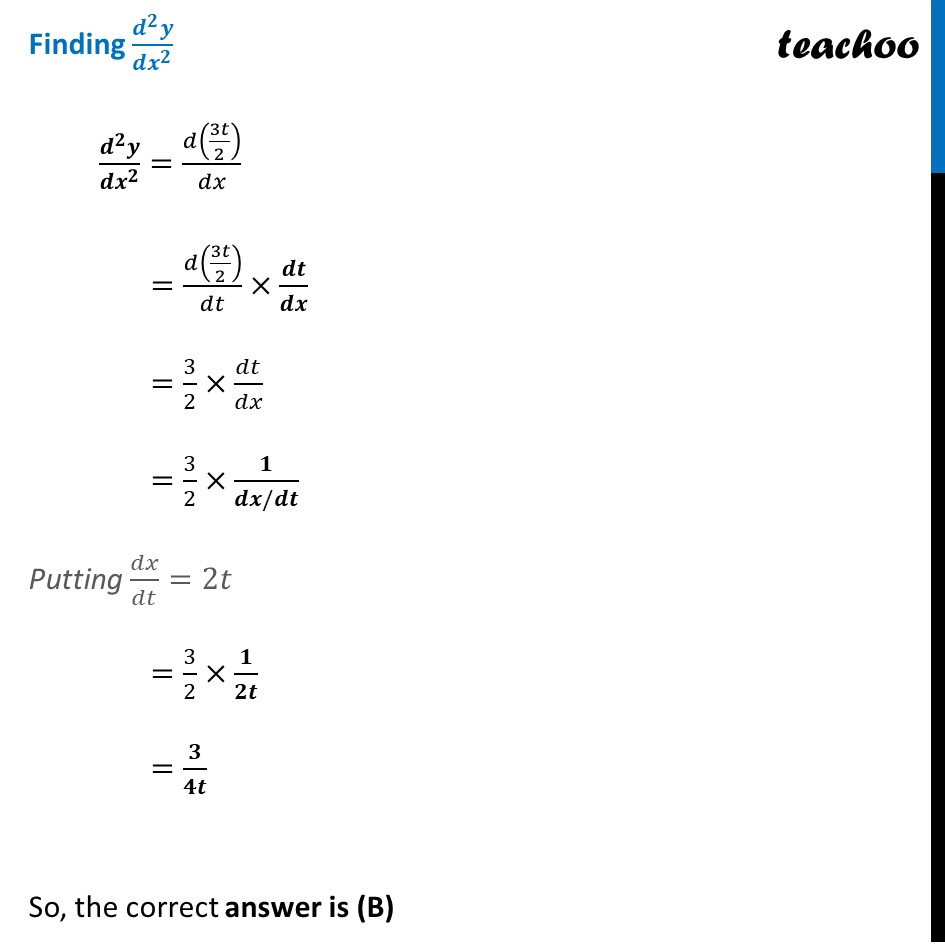NCERT Exemplar - MCQs

Chapter 5 Class 12 Continuity and Differentiability (Term 1)
Serial order wise

## (D) 3/4

This question is similar to Question 16 (Or 2nd) - CBSE Class 12 - Sample Paper for 2019 Boards - Solutions of Sample Papers and Past Year Papers - for Class 12 Boards### Transcript

Question 24 If x = t2, y = t3, then (𝑑^2 𝑦)/(𝑑𝑥^2 ) is (A) 3/2 (B) 3/4𝑡 (C) 3/2𝑡 (D) 3/4 𝑥=𝑡^2 & 𝑦=𝑡^3 Finding 𝒅𝒚/𝒅𝒙 𝒅𝒚/𝒅𝒙=(𝒅𝒚/𝒅𝒕)/(𝒅𝒙/𝒅𝒕) Finding 𝒅𝒚/𝒅𝒕 𝑦=𝑡^3 𝑑𝑦/𝑑𝑡=(𝑑(𝑡^3))/𝑑𝑡 𝒅𝒚/𝒅𝒕=𝟑𝒕^𝟐 Finding 𝒅𝒙/𝒅𝒕 𝑥 =𝑡^2 𝑑𝑥/𝑑𝑡=(𝑑(𝑡^2))/𝑑𝑡 𝒅𝒙/𝒅𝒕=𝟐𝒕 Now, 𝒅𝒚/𝒅𝒙=(𝒅𝒚/𝒅𝒕)/(𝒅𝒙/𝒅𝒕) =(3𝑡^2)/2𝑡 =𝟑𝒕/𝟐 Finding (𝒅^𝟐 𝒚)/(𝒅𝒙^𝟐 ) (𝒅^𝟐 𝒚)/(𝒅𝒙^𝟐 )=𝑑(3𝑡/2)/𝑑𝑥 =𝑑(3𝑡/2)/𝑑𝑡×𝒅𝒕/𝒅𝒙 =3/2×𝑑𝑡/𝑑𝑥 =3/2×𝟏/(𝒅𝒙/𝒅𝒕) Putting 𝑑𝑥/𝑑𝑡=2𝑡 =3/2×𝟏/𝟐𝒕 =𝟑/𝟒𝒕 So, the correct answer is (B)